Download PresentationChapter

# Chapter - PowerPoint PPT Presentation

Chapter. 8. Net Present Value and Other Investment Criteria. Key Concepts and Skills. Understand the payback rule and its shortcomings Understand accounting rates of return and their problems Understand the internal rate of return and its strengths and weaknessesI am the owner, or an agent authorized to act on behalf of the owner, of the copyrighted work described.
Download Presentation## Chapter

An Image/Link below is provided (as is) to download presentation

Download Policy: Content on the Website is provided to you AS IS for your information and personal use and may not be sold / licensed / shared on other websites without getting consent from its author.While downloading, if for some reason you are not able to download a presentation, the publisher may have deleted the file from their server.

- - - - - - - - - - - - - - - - - - - - - - - - - - E N D - - - - - - - - - - - - - - - - - - - - - - - - - -
Presentation TranscriptChapter

8

Net Present Value and Other Investment Criteria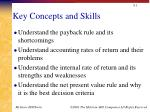Key Concepts and Skills
• Understand the payback rule and its shortcomings
• Understand accounting rates of return and their problems
• Understand the internal rate of return and its strengths and weaknesses
• Understand the net present value rule and why it is the best decision criteria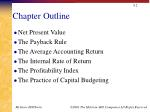Chapter Outline
• Net Present Value
• The Payback Rule
• The Average Accounting Return
• The Internal Rate of Return
• The Profitability Index
• The Practice of Capital BudgetingGood Decision Criteria
• We need to ask ourselves the following questions when evaluating decision criteria
• Does the decision rule adjust for the time value of money?
• Does the decision rule adjust for risk?
• Does the decision rule provide information on whether we are creating value for the firm?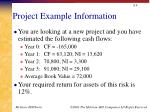Project Example Information
• You are looking at a new project and you have estimated the following cash flows:
• Year 0: CF = -165,000
• Year 1: CF = 63,120; NI = 13,620
• Year 2: 70,800; NI = 3,300
• Year 3: 91,080; NI = 29,100
• Average Book Value = 72,000
• Your required return for assets of this risk is 12%.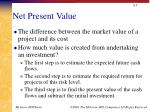Net Present Value
• The difference between the market value of a project and its cost
• How much value is created from undertaking an investment?
• The first step is to estimate the expected future cash flows.
• The second step is to estimate the required return for projects of this risk level.
• The third step is to find the present value of the cash flows and subtract the initial investment.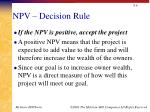NPV – Decision Rule
• If the NPV is positive, accept the project
• A positive NPV means that the project is expected to add value to the firm and will therefore increase the wealth of the owners.
• Since our goal is to increase owner wealth, NPV is a direct measure of how well this project will meet our goal.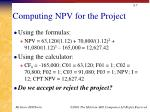Computing NPV for the Project
• Using the formulas:
• NPV = 63,120/(1.12) + 70,800/(1.12)2 + 91,080/(1.12)3 – 165,000 = 12,627.42
• Using the calculator:
• CF0 = -165,000; C01 = 63,120; F01 = 1; C02 = 70,800; F02 = 1; C03 = 91,080; F03 = 1; NPV; I = 12; CPT NPV = 12,627.42
• Do we accept or reject the project?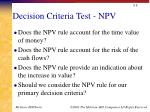Decision Criteria Test - NPV
• Does the NPV rule account for the time value of money?
• Does the NPV rule account for the risk of the cash flows?
• Does the NPV rule provide an indication about the increase in value?
• Should we consider the NPV rule for our primary decision criteria?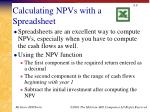Calculating NPVs with a Spreadsheet
• Spreadsheets are an excellent way to compute NPVs, especially when you have to compute the cash flows as well.
• Using the NPV function
• The first component is the required return entered as a decimal
• The second component is the range of cash flows beginning with year 1
• Subtract the initial investment after computing the NPVPayback Period
• How long does it take to get the initial cost back in a nominal sense?
• Computation
• Estimate the cash flows
• Subtract the future cash flows from the initial cost until the initial investment has been recovered
• Decision Rule – Accept if the payback period is less than some preset limitComputing Payback For The Project
• Assume we will accept the project if it pays back within two years.
• Year 1: 165,000 – 63,120 = 101,880 still to recover
• Year 2: 101,880 – 70,800 = 31,080 still to recover
• Year 3: 31,080 – 91,080 = -60,000 project pays back in year 3
• Do we accept or reject the project?Decision Criteria Test - Payback
• Does the payback rule account for the time value of money?
• Does the payback rule account for the risk of the cash flows?
• Does the payback rule provide an indication about the increase in value?
• Should we consider the payback rule for our primary decision criteria?Easy to understand

Adjusts for uncertainty of later cash flows

Biased towards liquidity

Ignores the time value of money

Requires an arbitrary cutoff point

Ignores cash flows beyond the cutoff date

Biased against long-term projects, such as research and development, and new projectsAverage Accounting Return
• There are many different definitions for average accounting return
• The one used in the book is:
• Average net income / average book value
• Note that the average book value depends on how the asset is depreciated.
• Need to have a target cutoff rate
• Decision Rule: Accept the project if the AAR is greater than a preset rate.Computing AAR For The Project
• Assume we require an average accounting return of 25%
• Average Net Income:
• (13,620 + 3,300 + 29,100) / 3 = 15,340
• AAR = 15,340 / 72,000 = .213 = 21.3%
• Do we accept or reject the project?Decision Criteria Test - AAR
• Does the AAR rule account for the time value of money?
• Does the AAR rule account for the risk of the cash flows?
• Does the AAR rule provide an indication about the increase in value?
• Should we consider the AAR rule for our primary decision criteria?Easy to calculate

Needed information will usually be available

Not a true rate of return; time value of money is ignored

Uses an arbitrary benchmark cutoff rate

Based on accounting net income and book values, not cash flows and market valuesInternal Rate of Return
• This is the most important alternative to NPV
• It is often used in practice and is intuitively appealing
• It is based entirely on the estimated cash flows and is independent of interest rates found elsewhereIRR – Definition and Decision Rule
• Definition: IRR is the return that makes the NPV = 0
• Decision Rule: Accept the project if the IRR is greater than the required returnComputing IRR For The Project
• If you do not have a financial calculator, then this becomes a trial and error process
• Calculator
• Enter the cash flows as you did with NPV
• Press IRR and then CPT
• IRR = 16.13% > 12% required return
• Do we accept or reject the project?Decision Criteria Test - IRR
• Does the IRR rule account for the time value of money?
• Does the IRR rule account for the risk of the cash flows?
• Does the IRR rule provide an indication about the increase in value?
• Should we consider the IRR rule for our primary decision criteria?• Knowing a return is intuitively appealing
• It is a simple way to communicate the value of a project to someone who doesn’t know all the estimation details
• If the IRR is high enough, you may not need to estimate a required return, which is often a difficult taskCalculating IRRs With A Spreadsheet
• You start with the cash flows the same as you did for the NPV
• You use the IRR function
• You first enter your range of cash flows, beginning with the initial cash flow
• You can enter a guess, but it is not necessary
• The default format is a whole percent – you will normally want to increase the decimal places to at least twoNPV Vs. IRR
• NPV and IRR will generally give us the same decision
• Exceptions
• Non-conventional cash flows – cash flow signs change more than once
• Mutually exclusive projects
• Initial investments are substantially different
• Timing of cash flows is substantially differentIRR and Non-conventional Cash Flows
• When the cash flows change sign more than once, there is more than one IRR
• When you solve for IRR you are solving for the root of an equation and when you cross the x-axis more than once, there will be more than one return that solves the equation
• If you have more than one IRR, which one do you use to make your decision?Another Example – Non-conventional Cash Flows
• Suppose an investment will cost \$90,000 initially and will generate the following cash flows:
• Year 1: 132,000
• Year 2: 100,000
• Year 3: -150,000
• The required return is 15%.
• Should we accept or reject the project?NPV Profile

IRR = 10.11% and 42.66%Summary of Decision Rules
• The NPV is positive at a required return of 15%, so you should Accept
• If you use the financial calculator, you would get an IRR of 10.11% which would tell you to Reject
• You need to recognize that there are non-conventional cash flows and look at the NPV profileIRR and Mutually Exclusive Projects
• Mutually exclusive projects
• If you choose one, you can’t choose the other
• Example: You can choose to attend graduate school next year at either Harvard or Stanford, but not both
• Intuitively you would use the following decision rules:
• NPV – choose the project with the higher NPV
• IRR – choose the project with the higher IRRExample With Mutually Exclusive Projects

The required return for both projects is 10%.

Which project should you accept and why?NPV Profiles

IRR for A = 19.43%

IRR for B = 22.17%

Crossover Point = 11.8%Conflicts Between NPV and IRR
• NPV directly measures the increase in value to the firm
• Whenever there is a conflict between NPV and another decision rule, you should always use NPV
• IRR is unreliable in the following situations
• Non-conventional cash flows
• Mutually exclusive projectsProfitability Index
• Measures the benefit per unit cost, based on the time value of money
• A profitability index of 1.1 implies that for every \$1 of investment, we create an additional \$0.10 in value
• This measure can be very useful in situations where we have limited capitalClosely related to NPV, generally leading to identical decisions

Easy to understand and communicate

May be useful when available investment funds are limited

May lead to incorrect decisions in comparisons of mutually exclusive investmentsCapital Budgeting In Practice
• We should consider several investment criteria when making decisions
• NPV and IRR are the most commonly used primary investment criteria
• Payback is a commonly used secondary investment criteriaQuick Quiz
• Consider an investment that costs \$100,000 and has a cash inflow of \$25,000 every year for 5 years. The required return is 9% and required payback is 4 years.
• What is the payback period?
• What is the NPV?
• What is the IRR?
• Should we accept the project?
• What decision rule should be the primary decision method?
• When is the IRR rule unreliable?